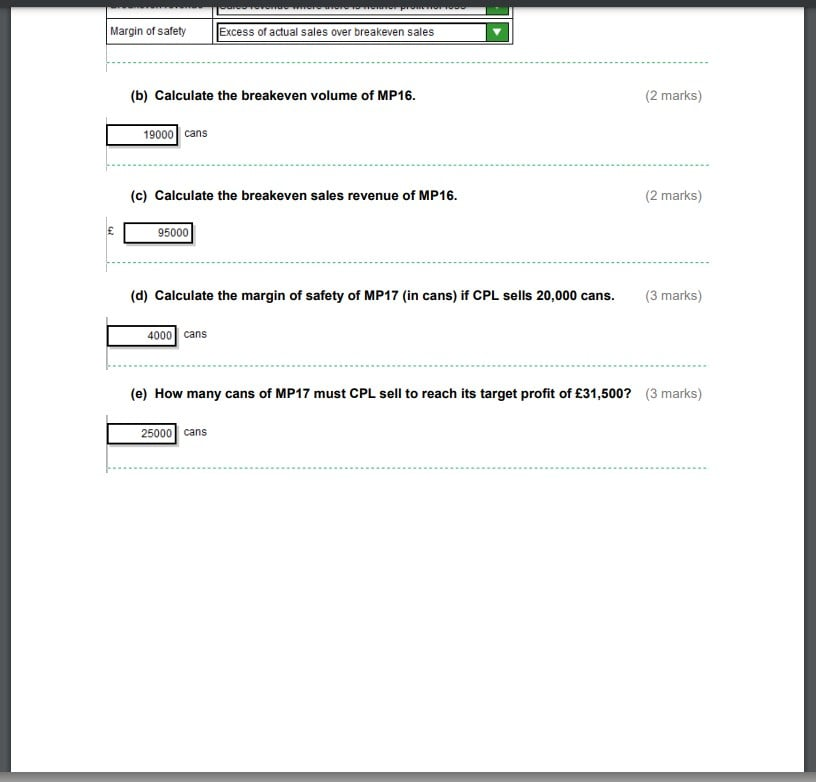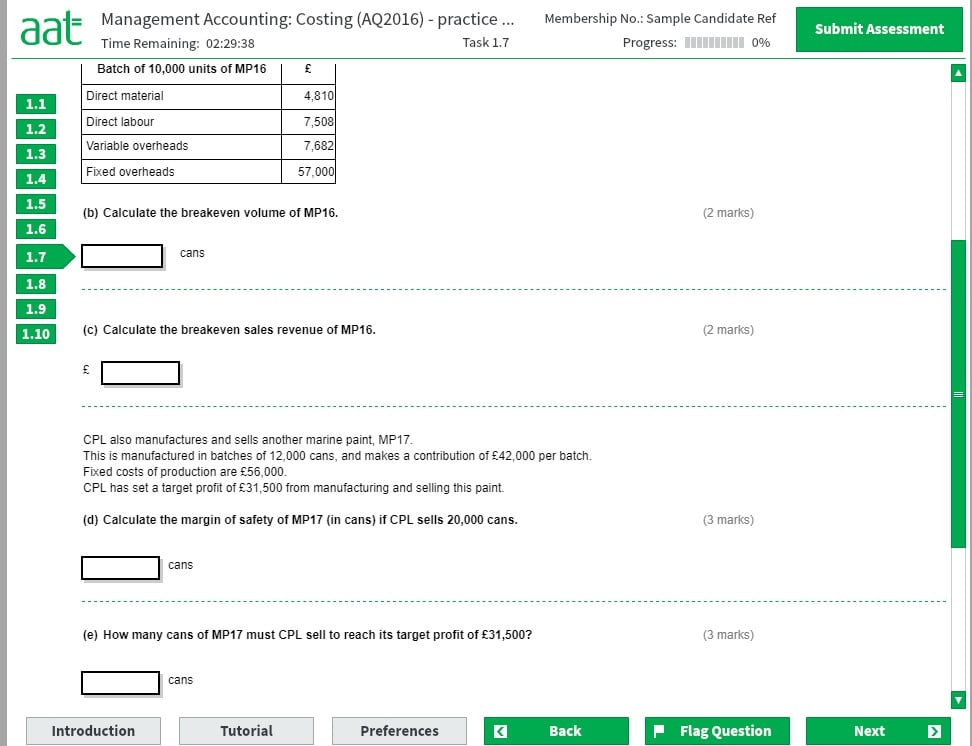# Management Accounting: Costing (AQ2016)- Practice Assessment 1 - 1.7

1.7
b) Breakeven volume = fixed costs / contribution per unit
(fixed costs)57000/(contribution per unit) ?
? = contribution is sales revenue less the variable costs
(sales revenue) 50000 (10000 cans £5.00 each) "less" - (contribution per unit) 20000 (Direct mat. 4810+ Direct labour 7508 + variable overheads 7682)=50000-20000=30000
Contribution per unit = 57000/30000=1.9 (can)
Break even Volume = 1.9*10000=19000 (per volume)

c) Breakeven Sales Revenue = 19,000 cans x selling price (£5.00) = Total revenue £95,000

d)Margin of safety (20000 cans)
Margin of safety = Budgeted sales units - brake even, = 20000- ?
? = brake even = fixed costs/ contribution per unit (42000 (contribution)/12000 (units))= 56000/3.5=16000
Margin of safety = 20000-16000=4000 units

e) how many cans to sell to reach its target profit
=(fixed costs + target profit)/contribution per unit
= (56000+31500)/3.5
=87500/3.5
=25000 cans